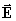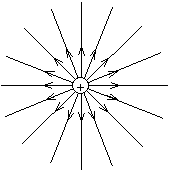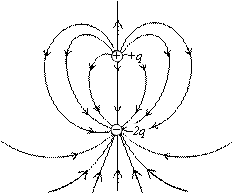# Physics Assignment Help With Electric Lines of Force

## 10.7 Electric Lines of Force

The lines of forces are purely geometrical construction which helps in visualising the nature of electric field in a region. It has no physical existence. Lines of forces are drawn in such a way that the tangent to a line of force gives the direction of resultant electric field. The density of field lines in any region is proportional to the magnitude of the electric field in that region. Field lines originate on positive charges and terminate on negative charges.

(1) There cannot be any closed line of force in an electrostatic field. Electric lines of force emerge from positive charges and terminate on negative charges (or extends to infinity).Fig. (A) Fig. (B)

(2) Crowded lines represent strong field, while distant weak field.

(3) The number of lines originating or terminating on a charge is proportional to the magnitude of the charge. In SI units, the number of electric field lines associated with a unit charge (i.e., 1 coulomb) is taken as 1/e0. Thus, if a body encloses a charge q, total lines of force associated with it (also called flux) will be q/e0.

Note that the total lines of force may be fractional as lines of force are imaginary.Electric field lines of two unequal charges of opposite sign

(4) Lines of force can never cross each other. It is obvious, because if they cross at a point the intensity at that point will have two directions, which is absurd.

(5) Lines of force have tendency to contract longitudinally (like a stretched elastic string) and repel each other laterally. This concept, like the lines of force, is also imaginary. It helps in understanding how attraction is produced between opposite charges, and how repulsion is produced between similar charges.

(6) If there is no electric field in a region of space, there will be no lines of force. This is why inside a conductor or at a neutral point (where resultant intensity is zero) there cannot be any line of force.

(7) If the lines of force are equidistant straight lines, the field is uniform [Fig. (A)]. If either lines of force are not equidistant or straight lines or both, the field will be non-uniform [Fig. (B), (C) and (D)].(A) (B) (C)

Uniform Field Non-uniform fields### Email Based Assignment Help in Electric Lines of Force

We are the leading online Assignment Help provider. Find answers to all of your doubts regarding the Electric Lines of Force. Assignmenthelp.net provide homework, Assignment Help to the school, college or university level students. Our expert online tutors are available to help you in Electric Lines of Force. Our service is focused on: time delivery, superior quality, creativity and originality.